This video was brought to you by GeneralPAC.com, making power systems Intuitive, Open and Free for Everyone, Everywhere. Consider subscribing and supporting through patreon.com/GeneralPAC. This is a mechanism for you to support us financially so we can continue making high quality power system video tutorials. Our corporate sponsor for this topic is AllumiaX.com from Seattle, Washington. Contact them for industrial and commercial power system studies.

In the previous video, Part 1b, we discussed about the per unit formula and the base values and solved a very small part of an example. In this video, Part 1c, we will complete Part (a) and Part (b) of that same example.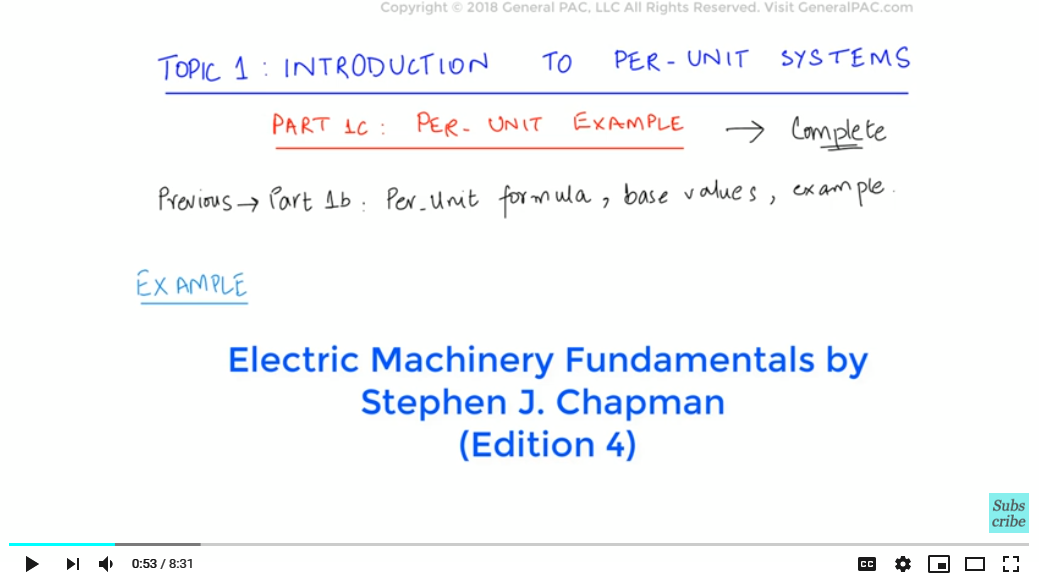The example is taken from a book called Electric Machinery Fundamentals by Stephan Chapman (Edition no. 4).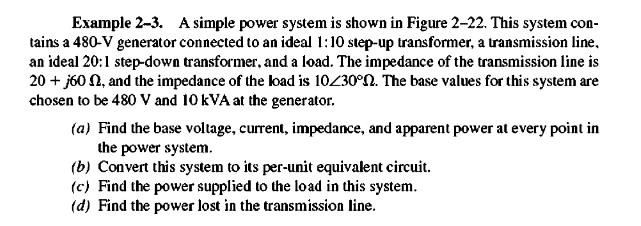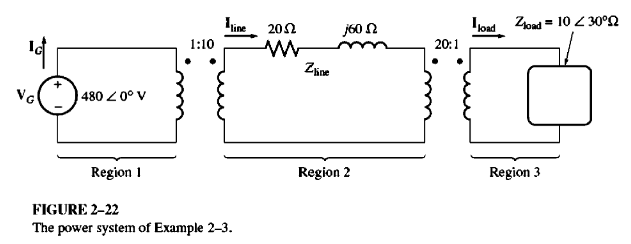Example 2.3, pg 95, Electric Machinery Fundamentals by Stephan J. Chapman (Edition 4)

We will not be solving Parts (c) and (d) since our objective is to focus on per part of this problem.. Part (a) Finding out base values for the different regions

Region 1

We solved this in our previous video, Part 1b. For Region 1, we calculated the base values for Ibase and Zbase using the given values of Vbase and Sbase. The answers were:

Ibase = 20.83 A

Zbase = 23.04 Ω

Region 2

First, let’s write down the base values that are given for Region 2. We know that the ratings of the generator both as Vbase and Sbase for region 1.. Since there is a step-up transformer between region 1 and region 2, the Vbase for Region 2 would be different however, the Sbase will remain same… in fact, the Sbase will remain the same for the entire system. So, for Region 2,

S(2,base) = 10 kVA (which will remain the same for the entire system)

Now let’s find out the base voltage by using the transformer turns ratio, we can simply find out the voltage on the secondary side of the transformer.

Vp / Vs
=
Np / Ns

Since

Vp = V(1,base) = 480 V

So:

480 V / Vs
=
1 / 10

Rearranging and solving for Vs:

Vs = 4800 V

And we know that this is the base voltage for Region 2 so:

V(2,base) = 4800 V

Calculating other base values:

I(2,base)
S(2,base) / V(2,base)
=
10 KVA / 4800 V
=2.083 A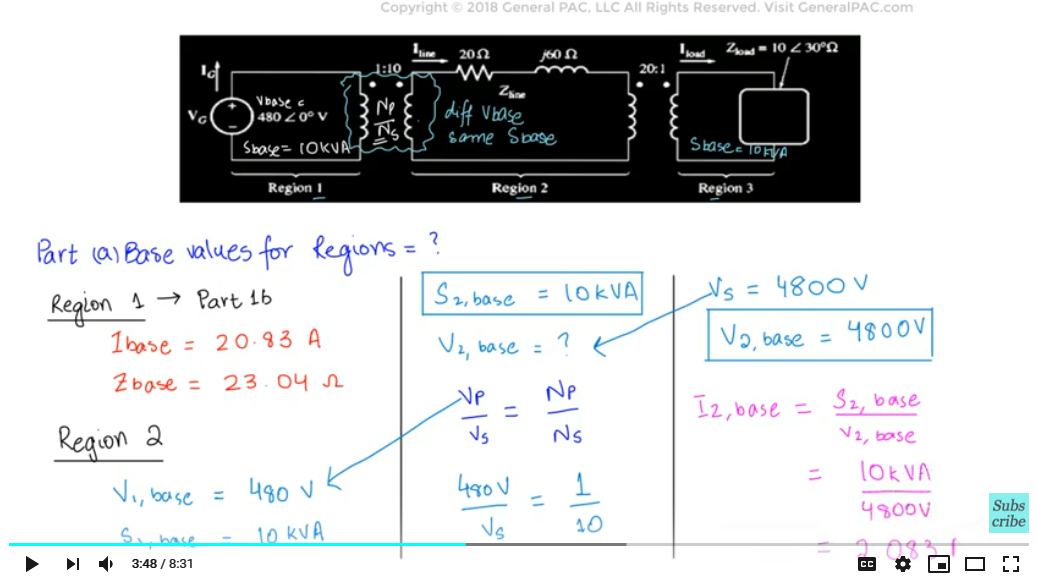Z(2,base)
V(2,base) / I(2,base)
=
4800 V / 2.083 A
=2304 Ω

We can also calculate these values using transformer turns ratio formulas.

Region 3

The base power is same throughout the system so:

S(3,base) = 10 kVA

Since there is a step-down transformer linking Region 2 and Region 3, so we will employ the calculation process as we did in Region 2 calculation.

V p / Vs
=
N p / N s

Vp = V(2,base) = 4800 V

4800 V / Vs
=
20 / 1

Vs = 240 V

V(3,base) = 240 V

Calculating other base values:

I(3,base) =
S(3,base) / V(3,base)
=
10 KVA / 240 V
= 41.67 A
z(3,base) =
V(3,base) / I(3,base)
=
240 V / 41.67 A
= 5.76 Ω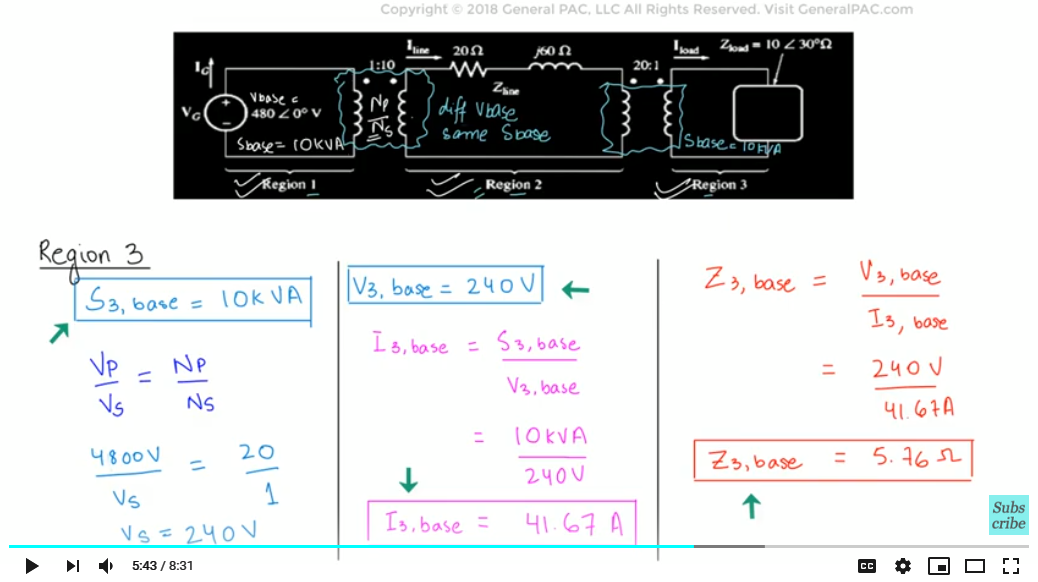We can also calculate these values using transformer turns ratio formulas.

Now that we have completed part b of the problem by caluating the base values for every region of the power system, let’s move on to the next part.

Part (b) Converting into per unit equivalent

The per-unit equivalent circuit consists of the components of individual regions. Region 1 is the generating region, so the equivalent would be the source. Region 2 is the transmission line, so the equivalent would be the per-unit impedance of the transmission line. Region 3 consists of load, so the equivalent circuit would contain per-unit impedance of the load. We know that the generic formula to find out per-unit values is:

Per unit value=
Actual value / Base value

Region 1

We calculated Vpu in the video Part 1b that was:

V(1,p.u) = 1.0 p.u

Region 2

We will calculate the per unit impedance of the transmission line:

z(2,p.u) =
Z(2,actual) / Z(2,base)
=
20 + j60 Ω / 2304 Ω
= 0.0087 + j0.0260 p.u

Region 3

We will calculate the per unit impedance of the load connected to the power system:

z(3,p.u) =
Z(3,actual) / Z(3,base)
=
10 ∠ 30° Ω / 5.76 Ω
= 1.736 ∠ 30° p.u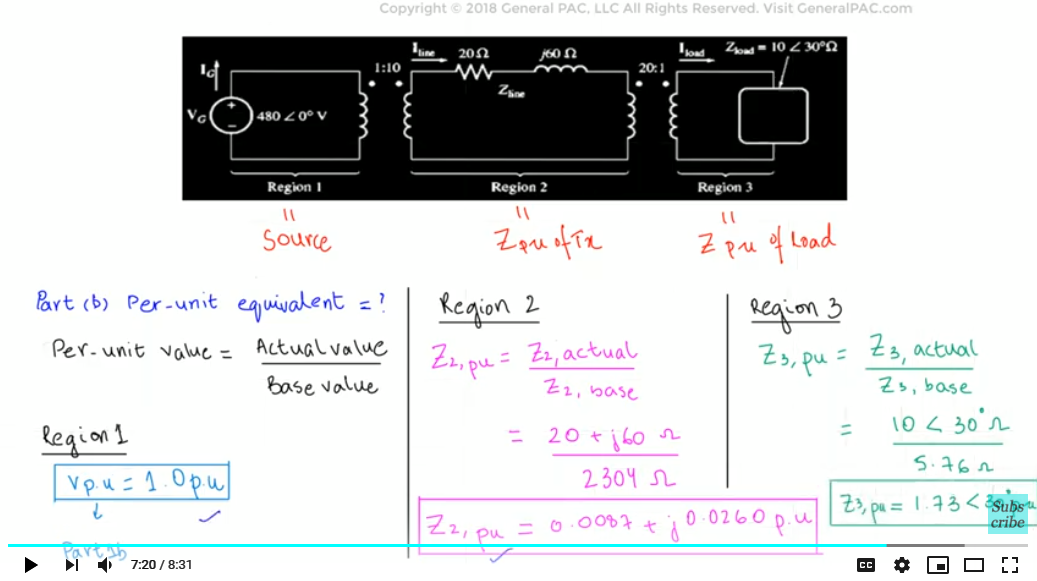Now since we have calculated the per-unit values, we will draw the equivalent circuit.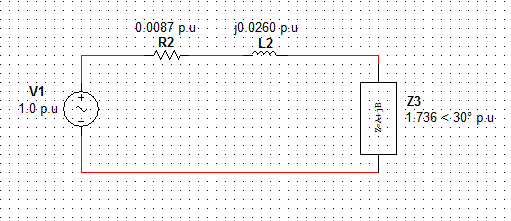As you can see, the per unit equivalent circuit is more simple because we have eliminated the different voltage levels completely and thereby eliminated region 1, 2, and 3 boundaries. This simplification is one of the biggest reasons why we use per unit in power systems. In the next video we will look into the per-unit formulas for single phase and three phase systems.

We hope, you have a continued interest in this topic and series as a student or professional. We also hope you find this content useful and enlightening. Please consider subscribing to GeneralPac.com or becoming a patron on patreon.com/generalpac.

GeneralPAC.com. We make high-quality Power Systems Video Tutorials on complex topics that are free and open to everyone!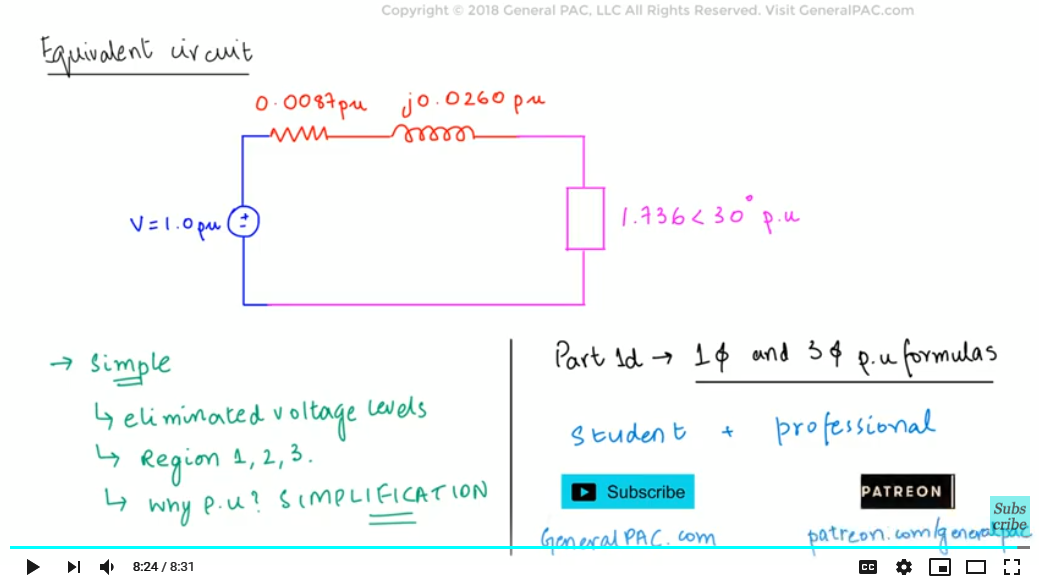#### Greetings from the GeneralPAC Team!

We make high-quality Power Systems Video Tutorials on complex topics that are free and open to everyone!  Thank you so much for supporting us through Patreon so we can continue doing good and valuable work.

What is Patreon and why do we use it?

Patreon is a fantastic portal that allows our fans and community to make monthly contribution (like Netflix subscription) so we can continue creating high-quality power systems video tutorials. In return, you get access to incredible perks like voting on future topics, getting your questions answered, access to VIP Q/A webinars with the creators of GeneralPAC, and much more! We THANK YOU for supporting us

Why do we need your support?

An incredible amount of time and effort is needed to develop high-quality video tutorials. Each video (Part 1 for example) takes approximately 10 hours to complete which includes learning the concept ourselves, brainstorming creative ways to teach and explain the concepts, writing the script, audio recording, video recording, and editing. It's no wonder why Hundreds-of-Thousands of people have watched, liked, subscribed, and left positive comments on Youtube channel. Your support truly makes all the difference.